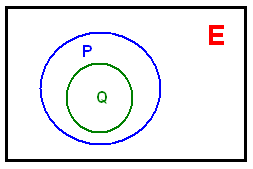# Crisp Sets Explained

A set is a well defined collection of objects. Here the word well defined means the object either belongs to or doesn’t belongs to the set. Observe the crispness in the example definitions below:

• A = { Firefox, Internet Explorer, Chrome }
• B = { Blogger, WordPress, TypePad }

VENN DIAGRAM is the pictorial representation to denote a set.Here,

• E is called universal set (or) universe of discourse.
• A is a set contained in the universal set E.

### Universe of Discourse

It is a set which contains all possible elements with similar characteristics with reference to a particular context. With the elements of this set, any number of sets can be formed.
The features of the elements in E can be discrete, countable integers or continuous valued quantities on real line.

#### Examples of Universal Set:

• The clock speed of computer processor
• The operating currents of an electric motor.
• The operation temperature of heat pump.
• The magnitude on Richter scale of an earthquake.

### Membership of Elements

An element ‘X’ is said to be a member of set A if X is contained in A or in other words it belongs to A. ( X ε A )

### Cardinality of a Set

The number of elements in a set is called its cardinality. Cardinality of a set A is denoted by n(A) or |A| or #A

### Family of Sets

A set whose members are sets themselves is referred to as family of sets.

A = { (1, 3, 5), (2, 4, 6), (5, 10, 15) }
Here (1, 3, 5), (2, 4, 6), (5, 10, 15) are individual sets contained in set A.

### Null Set or Empty Set

If a set ‘A’ has no elements in it or is empty then it called Null Set or Empty Set. The cardinality of a null set is zero.

n(A) = 0

### Singleton Set

Any set with a single element contained in it is called singleton set. The cardinality of such a set it 1.

|A| = 1

### SubsetGiven two sets ‘A’ and ‘B’ defined over universal set ‘E’. ‘A’ is said be a subset of ‘B’, if ‘A’ is fully contained in ‘B’. It is represented by A⊂B. When ‘A’ is equivalent or subset of B, then it is represented by A⊆B.

### SupersetGiven two sets ‘P’ and ‘Q’ defined on ‘E’ universe of discourse. ‘P’ is said to be a superset of ‘Q’, if ever element in ‘Q’ is contained in ‘P’. It is represented by P ⊃ Q.

### Powerset

Powerset of ‘A’ is the set of all possible subsets that are derivable from set A including null set. Powerset is represented by P(A). The cardinality of P(A) is 2|A|

For A = {2010, 2011} whose cardinality is |A| = 2
P(A)= { (2010), (2011), (2010, 2011), ( ) }

### What is a Countable Set and Uncountable Set

#### Countable Set

S is a given set, if we can define a one-one mapping from S to the set of natural numbers N. Then S is called as a countable set.

For example :-

1. Every finite set is a countable set.
2. Set of integers is a countable set.
3. Set of rational numbers is a countable set.

#### Uncountable Set

A set which is not countable which implies there cannot be a one-one mapping possible from the given set to set of natural numbers then that set is called an uncountable set.